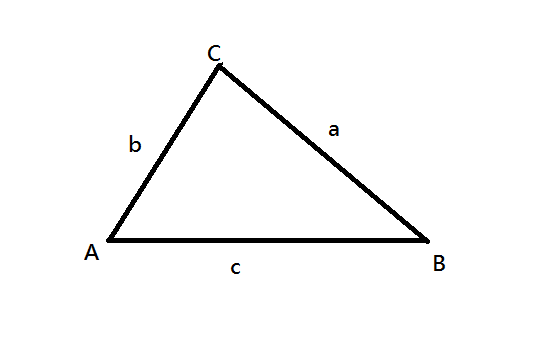# 三角形

• 直角三角形：Right-angled triangle 或 right triangle。
• 等腰三角形：Isosceles triangle，其中
• 兩腰的英文是 legs，第三邊稱為底 base。
• 兩腰的對角稱為底角，底角相等：Two angles opposite the legs (base angles) are equal.
• 兩腰的夾角稱為頂角 (vertex angle 或 apex angle)；頂角可能為銳角、直角或鈍角，但底角必為銳角。頂角的頂點稱為 apex。
• 底的中線 (median to the base 或者說 median from the apex，即頂點與底邊中點決定的直線）是等腰三角形的對稱軸 (axis of symmetry)； 此中線是頂角的角平分線 (the angle bisector of the vertex angle)， 也是底的中垂線 (the perpendicular bisector of the base)。
• 等邊三角形：Equilateral triangle，又稱為正三角形 (regular triangle)。
• 有時候將正三角形視為等腰三角形的特例，有時候規定正三角形並非等腰三角形，這件事並無全球的共識，要注意個別文件的前提。
• 銳角三角形：Acute-angled triangle（三角皆為銳角）。
• 鈍角三角形：Obtuse-angled triangle（某一角為鈍角）。
• 斜三角形：Oblique triangle（非直角三角形，銳角或鈍角三角形）又稱 non-right triangle。
• 不規則三角形：Scalene triangle（又稱為不等邊三角形，三邊不互等，可推論三角亦不互等）。我們習慣用 $$A$$, $$B$$, $$C$$ 表示三角形的頂點， 記作 $$\triangle ABC$$，讀作「三角形 ABC」(Triangle ABC)。 我們同時也用 $$A$$, $$B$$, $$C$$ 表示內角， 例如頂點 $$A$$ 的內角記作 $$\angle A$$， 讀作「角 A」(angle A)。 我們用 $$a$$, $$b$$, $$c$$ 同時表示邊和邊長， 而 $$a$$ 邊是角 $$A$$ 的對邊：Side $$a$$ is the side opposite angle $$A$$。

Sum of all the interior angles of a triangle is equal to a straight angle.

The exterior angle of a triangle is equal to the sum of the / two remote / opposite interior angles.

 [語音講解：triangle.mp3]

[ 回上層 ]

Created: Sep 5, 2005
Last Revised: 2022/02/28, 07/20,22, 11/06, 23/10/17